TensorSpace.jsTensorSpace Converter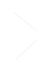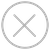TensorSpace.js Hello World

``````\$ tensorspacejs_converter \
--input_model_from="tensorflow" \
--input_model_format="tf_keras" \
./PATH/TO/MODEL/tf_keras_model.h5 \
./PATH/TO/SAVE/DIR``````
wb_sunny注意：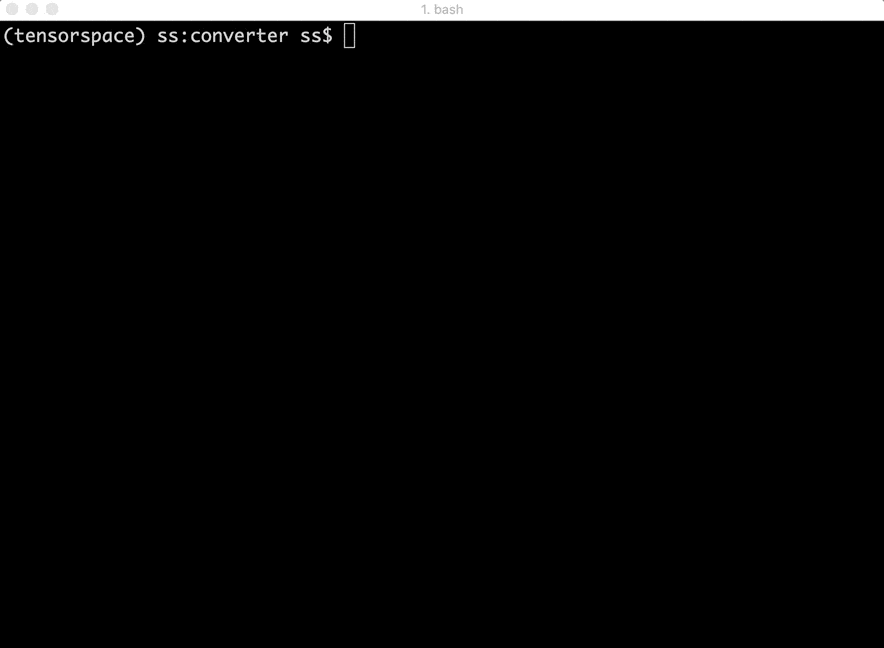``````let container = document.getElementById( "container" );
let model = new TSP.models.Sequential( container );``````

``````model.add( new TSP.layers.GreyscaleInput() );
outputs: ["0", "1", "2", "3", "4", "5", "6", "7", "8", "9"]
}) );``````

``````model.load({
type: "tensorflow",
url: './PATH/TO/MODEL/model.json'
});
model.init(function() {
console.log("Hello World from TensorSpace"!);
});``````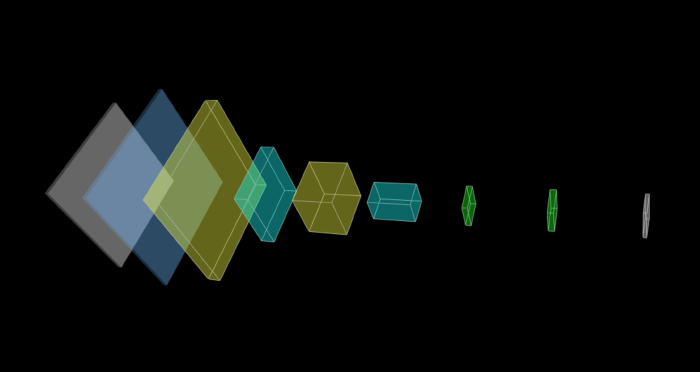``````model.init(function() {
model.predict( image_5 );
});``````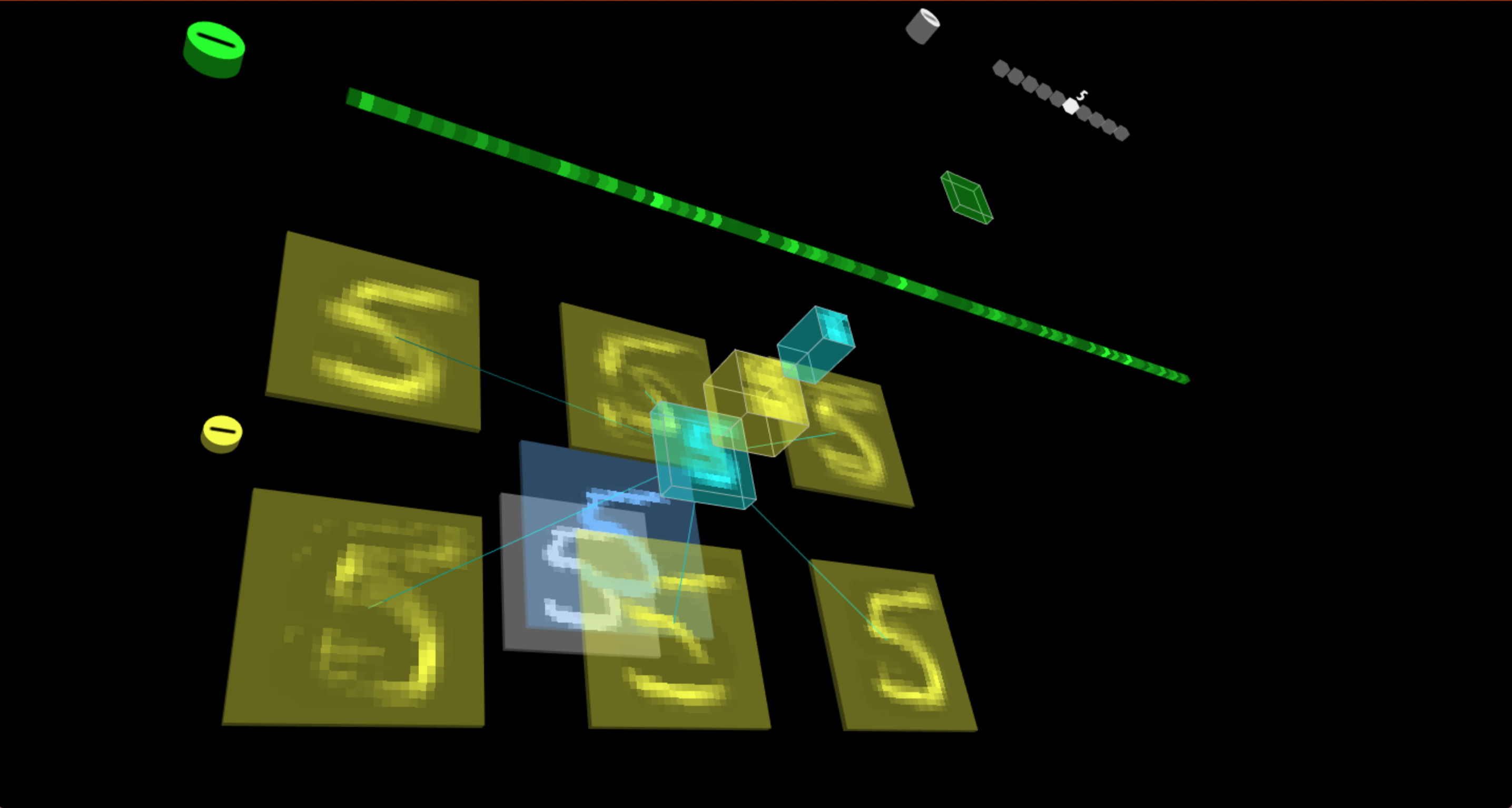TensorSpace 在线 CodePen 编辑：

See the Pen 667a7943b0f23727790ca38c93389689 by syt123450 (@syt123450) on CodePen.